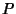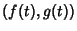## Pedal Curve

Given a curve, the pedal curve ofwith respect to a fixed point(the Pedal Point) is the locus of the pointof intersection of the Perpendicular fromto a Tangent to. The parametric equations for a curverelative to the Pedal PointareCurve Pedal Point Pedal Curve Astroid center Quadrifolium Cardioid cusp Cayley's Sextic Central Conic Focus Circle Circle any point Limaçon Circle on Circumference Cardioid Circle Involute center of Circle Archimedean Spiral Cissoid of Diocles Focus Cardioid Deltoid center Trifolium Deltoid cusp simple Folium Deltoid on curve unsymmetric double folium Deltoid Vertex double folium Epicycloid center Rose Hypocycloid center Rose Line any point point Logarithmic Spiral pole Logarithmic Spiral Parabola Focus Line Parabola foot of Directrix Right Strophoid Parabola on Directrix Strophoid Parabola reflection of Focus by Directrix Maclaurin Trisectrix Parabola Vertex Cissoid of Diocles Sinusoidal Spiral pole Sinusoidal Spiral Tschirnhausen Cubic Focus of Pedal Parabola

References

Lawrence, J. D. A Catalog of Special Plane Curves. New York: Dover, pp. 46-49 and 204, 1972.

Lee, X. Pedal.'' http://www.best.com/~xah/SpecialPlaneCurves_dir/Pedal_dir/pedal.html.

Lockwood, E. H. Pedal Curves.'' Ch. 18 in A Book of Curves. Cambridge, England: Cambridge University Press, pp. 152-155, 1967.

Yates, R. C. Pedal Curves.'' A Handbook on Curves and Their Properties. Ann Arbor, MI: J. W. Edwards, pp. 160-165, 1952.